# Logistic Regression¶

Logistic regression estimates a linear relationship between a set of features and a binary outcome (usually $1$ for yes and $0$ for no), mediated by a sigmoid function to ensure the model produces probabilities. The frequentist approach results in point estimates for the parameters that measure the influence of each feature on the probability that a data point belongs to the positive or negative class.

## Logistic Function¶

The logistic function, also called the sigmoid function is an S-shaped curve that can take any real-valued number and map it into a value between $0$ and $1$ representing probability.

$$f(x)={\frac {1}{1+e^{-k(x-x_{0})}}}$$

where

• $x_{0} =$ the $x$ value of the sigmoid's midpoint,
• $k =$ the logistic growth rate or steepness of the curve,
• $e$ is the base of the natural logarithms or the np.exp() function.

### Representation Used for Logistic Regression¶

Logistic regression uses an equation as the representation, very much like linear regression.

Input values $(x)$ are combined linearly using weights or coefficient values (referred to as the Greek capital letter Beta $\beta$) to predict an output value $(y)$. A key difference from linear regression is that the output value being modeled is a binary values ($0$ or $1$) rather than a numeric value.

Example logistic regression equation:

$$y = \frac{e^{(\beta_0 + \beta_1*x)}}{(1 + e^{(\beta_0 + \beta_1*x)})}$$

Where $y$ is the predicted output, $\beta_0$ is the bias or intercept term and $\beta_1$ is the coefficient for the single input value $(x)$. Each column in your input data has an associated $\beta$ coefficient (a constant real value) that must be learned from the training data.

In :
import warnings
warnings.filterwarnings('ignore')

In :
import pandas as pd
import numpy as np
import statsmodels.api as sm
import matplotlib.pyplot as plt
import seaborn as sns

In :
sns.set(style="darkgrid", color_codes=True)
pd.set_option('display.max_columns', None)


Here we define a sigmoid function and plot it for a range of values between $-10$ and $10$

In :
def sigmoid(x):
return 1 / (1 + np.exp(-x))

In :
x = np.arange(-10,11,1)
y = sigmoid(x)

plt.plot(x,y)
plt.xlabel('X')
plt.ylabel('Sigmoid(X)');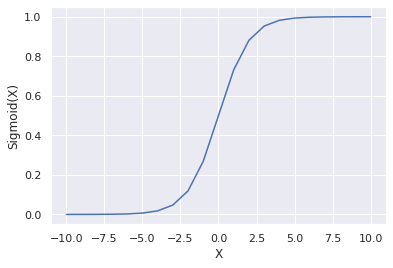## Logistic Regression Example¶

### Data Set¶

Quarterly US macro data from $1959 – 2009$

In :
data = pd.DataFrame(sm.datasets.macrodata.load().data)
data.info()

<class 'pandas.core.frame.DataFrame'>
RangeIndex: 203 entries, 0 to 202
Data columns (total 14 columns):
year        203 non-null float64
quarter     203 non-null float64
realgdp     203 non-null float64
realcons    203 non-null float64
realinv     203 non-null float64
realgovt    203 non-null float64
realdpi     203 non-null float64
cpi         203 non-null float64
m1          203 non-null float64
tbilrate    203 non-null float64
unemp       203 non-null float64
pop         203 non-null float64
infl        203 non-null float64
realint     203 non-null float64
dtypes: float64(14)
memory usage: 22.3 KB

In :
data.head()

Out:
year quarter realgdp realcons realinv realgovt realdpi cpi m1 tbilrate unemp pop infl realint
0 1959.0 1.0 2710.349 1707.4 286.898 470.045 1886.9 28.98 139.7 2.82 5.8 177.146 0.00 0.00
1 1959.0 2.0 2778.801 1733.7 310.859 481.301 1919.7 29.15 141.7 3.08 5.1 177.830 2.34 0.74
2 1959.0 3.0 2775.488 1751.8 289.226 491.260 1916.4 29.35 140.5 3.82 5.3 178.657 2.74 1.09
3 1959.0 4.0 2785.204 1753.7 299.356 484.052 1931.3 29.37 140.0 4.33 5.6 179.386 0.27 4.06
4 1960.0 1.0 2847.699 1770.5 331.722 462.199 1955.5 29.54 139.6 3.50 5.2 180.007 2.31 1.19

## Data Prep¶

To obtain a binary target variable, we compute the $20$-quarter rolling average of the annual growth rate of quarterly real GDP. We then assign $1$ if current growth exceeds the moving average and $0$ otherwise. Finally, we shift the indicator variables to align next quarter's outcome with the current quarter.

In :
data['growth_rate'] = data.realgdp.pct_change(4)
data['target'] = (data.growth_rate > data.growth_rate.rolling(20).mean()).astype(int).shift(-1)
data.quarter = data.quarter.astype(int)

In :
data.tail()

Out:
year quarter realgdp realcons realinv realgovt realdpi cpi m1 tbilrate unemp pop infl realint growth_rate target
198 2008.0 3 13324.600 9267.7 1990.693 991.551 9838.3 216.889 1474.7 1.17 6.0 305.270 -3.16 4.33 0.000262 0.0
199 2008.0 4 13141.920 9195.3 1857.661 1007.273 9920.4 212.174 1576.5 0.12 6.9 305.952 -8.79 8.91 -0.018619 0.0
200 2009.0 1 12925.410 9209.2 1558.494 996.287 9926.4 212.671 1592.8 0.22 8.1 306.547 0.94 -0.71 -0.033026 0.0
201 2009.0 2 12901.504 9189.0 1456.678 1023.528 10077.5 214.469 1653.6 0.18 9.2 307.226 3.37 -3.19 -0.038297 0.0
202 2009.0 3 12990.341 9256.0 1486.398 1044.088 10040.6 216.385 1673.9 0.12 9.6 308.013 3.56 -3.44 -0.025086 NaN
In :
pct_cols = ['realcons', 'realinv', 'realgovt', 'realdpi', 'm1', 'realgdp', 'pop', 'cpi', 'tbilrate', 'unemp']
drop_cols = ['year', 'growth_rate']

data.loc[:, pct_cols] = data.loc[:, pct_cols].pct_change(4)

In :
data = pd.get_dummies(data.drop(drop_cols, axis=1), columns=['quarter']).dropna()

In :
data.head()

Out:
realgdp realcons realinv realgovt realdpi cpi m1 tbilrate unemp pop infl realint target quarter_1 quarter_2 quarter_3 quarter_4
4 0.050676 0.036957 0.156237 -0.016692 0.036356 0.019324 -0.000716 0.241135 -0.103448 0.016151 2.31 1.19 0.0 1 0 0 0
5 0.020005 0.034147 -0.040877 -0.043426 0.024170 0.013722 -0.010586 -0.129870 0.019608 0.015976 0.14 2.55 0.0 0 1 0 0
6 0.022891 0.019409 0.024718 -0.033758 0.026821 0.013629 0.002847 -0.382199 0.056604 0.016070 2.70 -0.34 0.0 0 0 1 0
7 0.006252 0.019673 -0.132257 -0.015738 0.018278 0.016003 0.007857 -0.471132 0.125000 0.016172 1.21 1.08 0.0 0 0 0 1
8 -0.009985 0.009715 -0.196903 0.029544 0.014830 0.009140 0.017908 -0.322857 0.307692 0.016583 -0.40 2.77 0.0 1 0 0 0
In :
data.info()

<class 'pandas.core.frame.DataFrame'>
Int64Index: 198 entries, 4 to 201
Data columns (total 17 columns):
realgdp      198 non-null float64
realcons     198 non-null float64
realinv      198 non-null float64
realgovt     198 non-null float64
realdpi      198 non-null float64
cpi          198 non-null float64
m1           198 non-null float64
tbilrate     198 non-null float64
unemp        198 non-null float64
pop          198 non-null float64
infl         198 non-null float64
realint      198 non-null float64
target       198 non-null float64
quarter_1    198 non-null uint8
quarter_2    198 non-null uint8
quarter_3    198 non-null uint8
quarter_4    198 non-null uint8
dtypes: float64(13), uint8(4)
memory usage: 22.4 KB


## EDA¶

In :
total = len(data)
plt.figure(figsize=(7,5))
g = sns.countplot(x='target', data=data)
g.set_ylabel('Count', fontsize=14)
for p in g.patches:
height = p.get_height()
g.text(p.get_x()+p.get_width()/2.,
height + 1.5,
'{:1.2f}%'.format(height/total*100),
ha="center", fontsize=14, fontweight='bold')
plt.margins(y=0.1)
plt.show()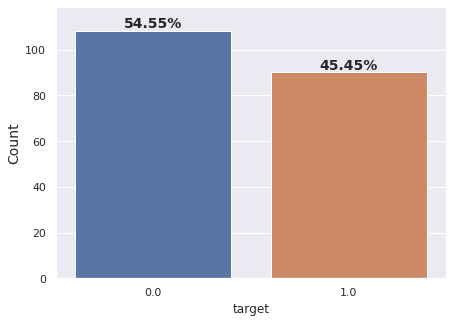In :
plt.figure(figsize=(16, 8))
corr = data.corr()
b, t = plt.ylim()
b += 0.5
t -= 0.5
plt.ylim(b, t)
plt.show()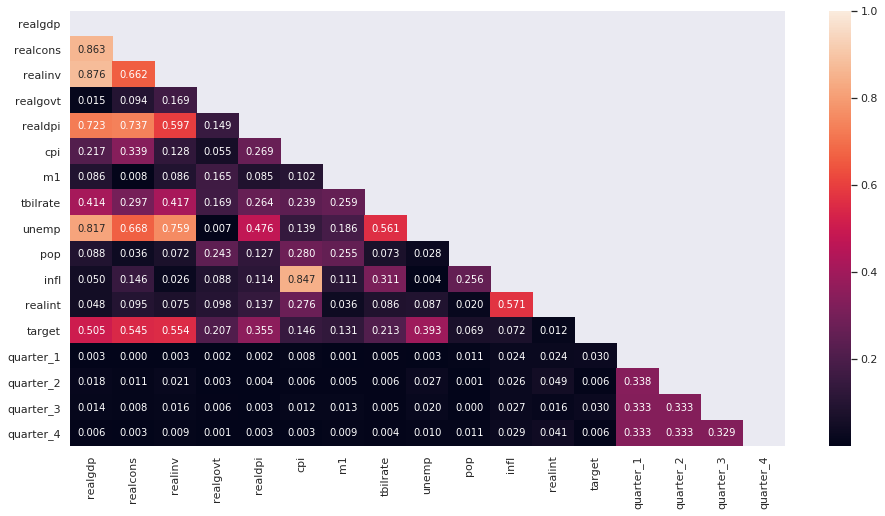In :
import matplotlib.cm as cm

n_fts = len(data.drop('target', axis=1).columns)
colors = cm.rainbow(np.linspace(0, 1, n_fts))

_ = data.drop('target', axis=1).corrwith(data.target).sort_values(ascending=True).plot(kind='barh',
color=colors, figsize=(10, 4))
plt.title('Correlation to Target')
plt.show()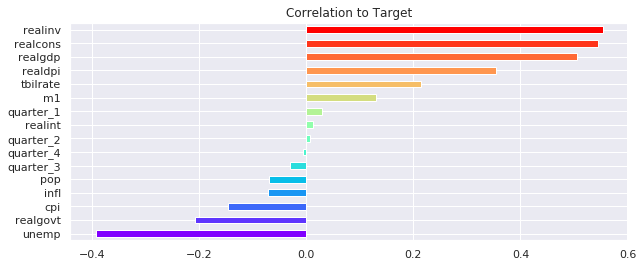In :
basetable0 = data.copy()

# Get all the variable names except "target"
variables = list(basetable0.columns)
variables.remove("target")

# Loop through all the variables and discretize in 5 bins if there are more than 5 different values
for variable in variables:
if len(basetable0.groupby(variable)) > 10:
new_variable = "disc_" + variable
basetable0[new_variable] = pd.qcut(basetable0[variable].round(4), 5)

# Print the columns in the new basetable
basetable0 = basetable0.drop(variables, axis=1)
print('\n', basetable0.columns)

 Index(['target', 'disc_realgdp', 'disc_realcons', 'disc_realinv',
'disc_realgovt', 'disc_realdpi', 'disc_cpi', 'disc_m1', 'disc_tbilrate',
'disc_unemp', 'disc_pop', 'disc_infl', 'disc_realint'],
dtype='object')

In :
# Function that creates predictor insight graph table
def create_pig_table(basetable, target, variable):

# Create groups for each variable
groups = basetable[[target,variable]].groupby(variable)

# Calculate size and target incidence for each group
pig_table = groups[target].agg(Incidence = np.mean, Size = np.size).reset_index()

# Return the predictor insight graph table
return pig_table

In :
# Variables you want to make predictor insight graph tables for
variables = list(basetable0.columns)
variables.remove("target")

# Create an empty dictionary
pig_tables = {}

# Loop through the variables
for variable in variables:

# Create a predictor insight graph table
pig_table = create_pig_table(basetable0, "target", variable)

# Add the table to the dictionary
pig_tables[variable] = pig_table

# Print the predictor insight graph table of the variable "disc_realgdp"
print(pig_tables["disc_realgdp"])

        disc_realgdp  Incidence  Size
0  (-0.0393, 0.0153]   0.050000  40.0
1    (0.0153, 0.029]   0.179487  39.0
2    (0.029, 0.0406]   0.675000  40.0
3   (0.0406, 0.0497]   0.666667  39.0
4   (0.0497, 0.0851]   0.700000  40.0

In :
# The function to plot a predictor insight graph
def plot_pig(pig_table, variable):

plt.figure(figsize=(10, 4))
# Plot formatting
plt.ylabel("Size", rotation=0, rotation_mode="anchor", ha="right")

# Plot the bars with sizes
pig_table["Size"].plot(kind="bar", width=0.5, color="pink", edgecolor="none", alpha=0.3)

# Plot the incidence line on secondary axis
pig_table["Incidence"].plot(secondary_y=True)

# Plot formatting
plt.xticks(np.arange(len(pig_table)), pig_table[variable])
plt.xlim([-0.5, len(pig_table) - 0.5])
plt.ylabel("Incidence", rotation=0, rotation_mode="anchor", ha="left")
plt.title(f'{variable}')

# Show the graph
plt.show()

In :
# Loop through the variables
for variable in variables:

# Create the predictor insight graph table
pig_table = create_pig_table(basetable0, "target", variable)

# Plot the predictor insight graph
plot_pig(pig_table, variable)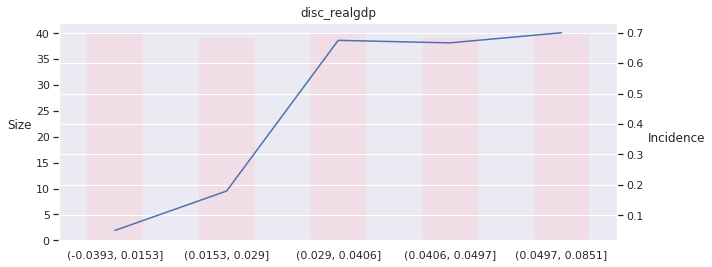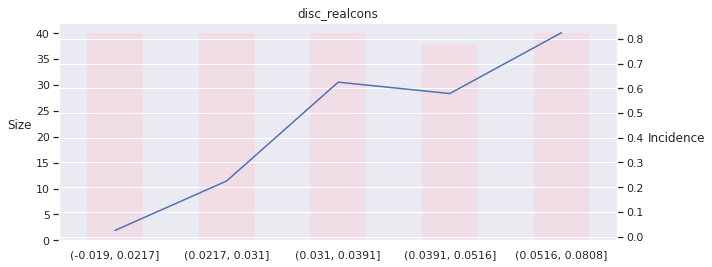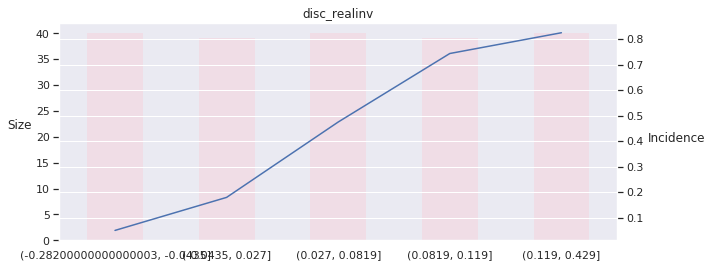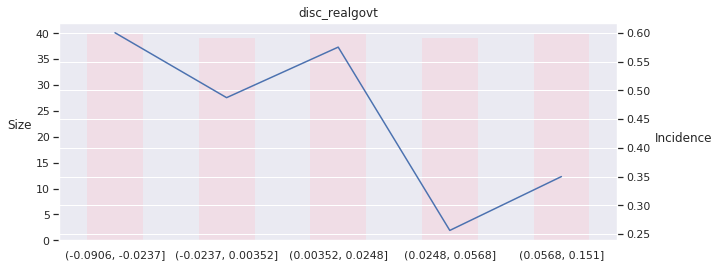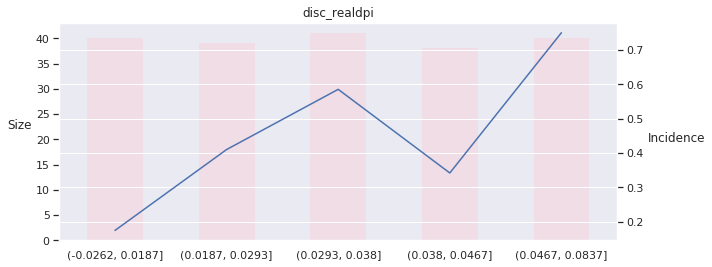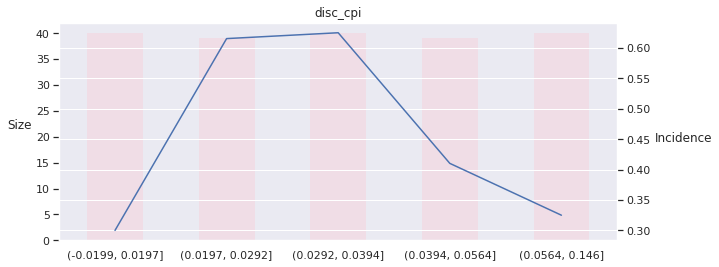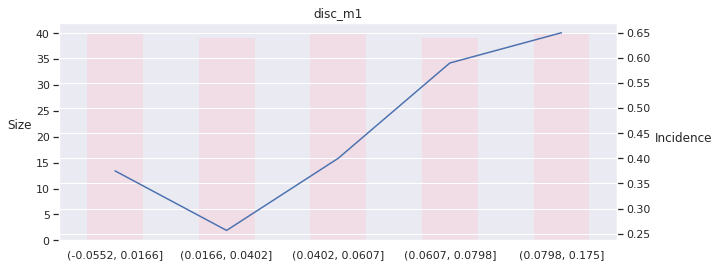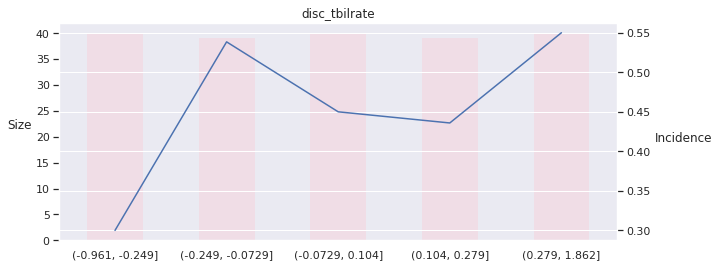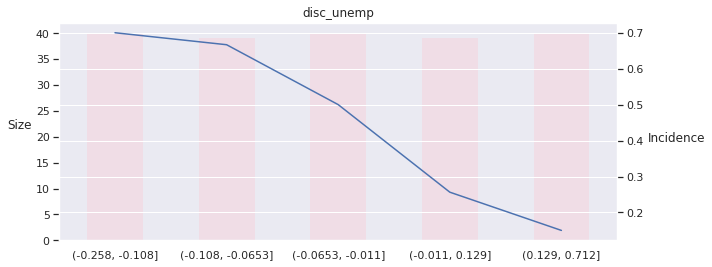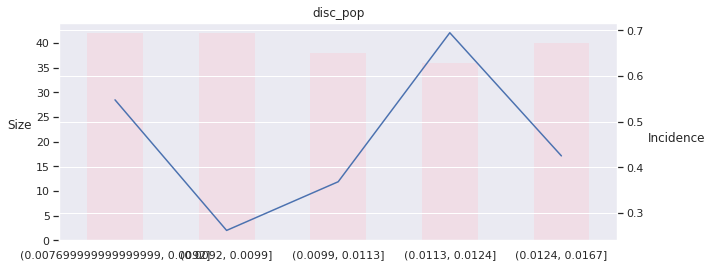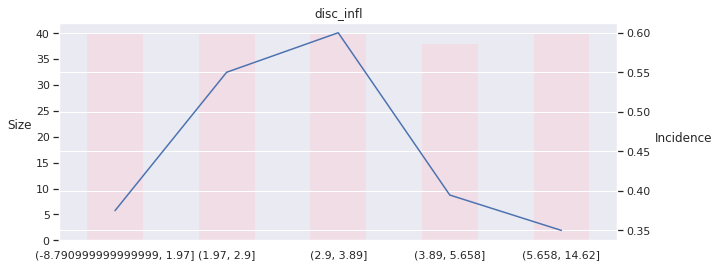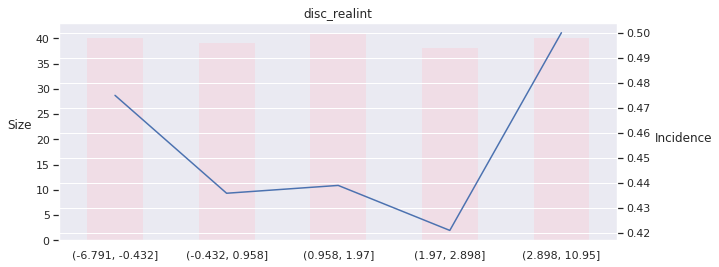## Fit Logistic Regression¶

We use an intercept and convert the quarter values to dummy variables and train the logistic regression model as follows:

In :
model = sm.Logit(data.target, sm.add_constant(data.drop('target', axis=1)))
result = model.fit()
result.summary()

Optimization terminated successfully.
Current function value: 0.335442
Iterations 9

Out:
Dep. Variable: No. Observations: target 198 Logit 182 MLE 15 Thu, 01 Oct 2020 0.5132 01:24:57 -66.417 True -136.42 nonrobust 2.288e-22
coef std err z P>|z| [0.025 0.975] 1.5416 6.83e+06 2.26e-07 1.000 -1.34e+07 1.34e+07 -84.1671 44.337 -1.898 0.058 -171.066 2.732 173.7999 39.838 4.363 0.000 95.719 251.880 35.2613 7.280 4.844 0.000 20.993 49.529 -11.9359 6.624 -1.802 0.072 -24.920 1.048 -42.5489 20.847 -2.041 0.041 -83.407 -1.690 -0.8881 27.725 -0.032 0.974 -55.228 53.451 7.7942 5.461 1.427 0.154 -2.910 18.498 0.0715 0.786 0.091 0.927 -1.469 1.612 4.4700 3.205 1.395 0.163 -1.813 10.753 -496.9396 178.376 -2.786 0.005 -846.550 -147.329 -0.1254 0.249 -0.503 0.615 -0.614 0.364 -0.0890 0.144 -0.619 0.536 -0.371 0.193 0.5723 6.83e+06 8.38e-08 1.000 -1.34e+07 1.34e+07 0.4601 6.83e+06 6.73e-08 1.000 -1.34e+07 1.34e+07 0.0408 6.83e+06 5.98e-09 1.000 -1.34e+07 1.34e+07 0.4683 6.83e+06 6.86e-08 1.000 -1.34e+07 1.34e+07

This produces the following summary for our model with $198$ observations and $16$ variables, including intercept: The summary indicates that the model has been trained using maximum likelihood and provides the maximized value of the log-likelihood function at $-66.4$.

• likelihood function: The likelihood function (often simply called the likelihood) measures the goodness of fit of a statistical model to a sample of data for given values of the unknown parameters. It is formed from the joint probability distribution of the sample, but viewed and used as a function of the parameters only, thus treating the random variables as fixed at the observed values.
• The likelihood function represents the combination of model parameter values that maximize the probability of drawing the sample obtained.The procedure for obtaining these arguments of the maximum of the likelihood function is known as maximum likelihood estimation, which for computational convenience is usually done using the natural logarithm of the likelihood, known as the log-likelihood function.
• maximum likelihood estimation: Maximum likelihood estimation (MLE) is a method of estimating the parameters of a probability distribution by maximizing a likelihood function, so that under the assumed statistical model the observed data is most probable. The point in the parameter space that maximizes the likelihood function is called the maximum likelihood estimate.
In :
# Image of model summary
plt.rc('figure', figsize=(12, 7))
plt.text(0.01, 0.05, str(result.summary()), {'fontsize': 14}, fontproperties = 'monospace')
plt.axis('off')
plt.tight_layout()
plt.savefig('logistic_example.png', bbox_inches='tight', dpi=300);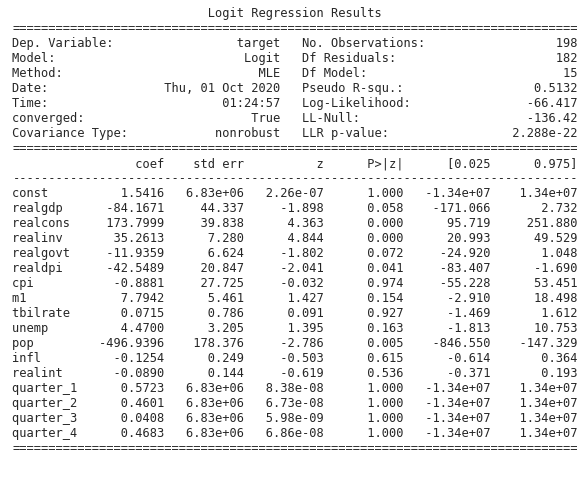In :
# Store p-values in dictionary
pvals = result.pvalues[1:].to_dict()
pvals

Out:
{'realgdp': 0.05765035302701731,
'realcons': 1.2846790085441199e-05,
'realinv': 1.2739780714173386e-06,
'realgovt': 0.071578784150557,
'realdpi': 0.041245807979824425,
'cpi': 0.9744466146149555,
'm1': 0.15354027960377567,
'tbilrate': 0.9274729258852897,
'unemp': 0.16316542882812857,
'pop': 0.005337771929708511,
'infl': 0.6151894399881822,
'realint': 0.5358600251228749,
'quarter_1': 0.9999999331604994,
'quarter_2': 0.9999999462713322,
'quarter_3': 0.9999999952311711,
'quarter_4': 0.9999999453030424}

The LL-Null value of $-136.42$ is the result of the maximized log-likelihood function when only an intercept is included. It forms the basis for the pseudo-R2 statistic and the Log-Likelihood Ratio (LLR) test. The pseudo-R2 statistic is a substitute for the familiar R2 available under least squares. It is computed based on the ratio of the maximized log-likelihood function for the null model $m_0$ and the full model $m_1$.

The values vary from $0$ (when the model does not improve the likelihood) to 1 where the model fits perfectly and the log-likelihood is maximized at $0$. Consequently, higher values indicate a better fit.

## Scikit Learn Example With Regularization¶

Regularization can be used to train models that generalize better on unseen data, by preventing the algorithm from overfitting the training dataset by penalizing the models coefficients.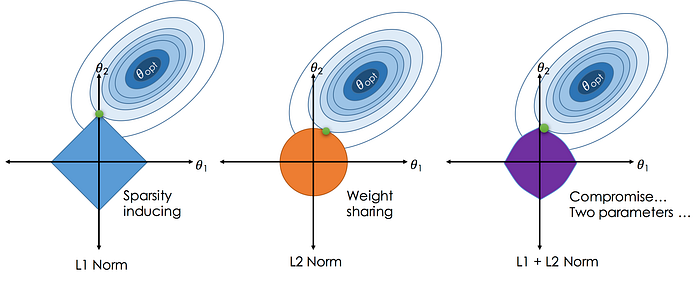Types of weight regularization:

• l1 (Lasso): Sum of the weights $$\lambda \Sigma_{i=1}^{k} |w_{i}|$$
• l2 (Ridge): Sum of the square of weights $$\lambda \Sigma_{i=1}^{k} w_{i}^{2}$$
• l1 + l2 (Elastic Net): Sum of the absolute and the squared weights.
$$\frac{\Sigma_{i=1}^{n}(y_{i} - x_{i}^{J} \hat{\beta})^{2}}{2n} + \lambda \left( \frac{1 - a}{2} \sum_{j=1}^{m} \hat{\beta_{j}^{2}} + a \sum_{j=1}^{m} |\hat{\beta_{j}}| \right)$$

### Logistic regression with different types of regularization¶

Here, we fit a logistic regression model for the various types of regularization and without regularization. Then we evaluate with 3-fold cross validation.

In :
from sklearn.linear_model import LogisticRegression
from sklearn.model_selection import cross_val_score, StratifiedKFold, train_test_split, TimeSeriesSplit
from sklearn.metrics import roc_auc_score

#cv = StratifiedKFold(3)
cv = TimeSeriesSplit(n_splits=3)

X, y = data.drop('target', axis=1), data.target
X_train, X_test, y_train, y_test = train_test_split(X, y, test_size=0.33, shuffle=False)

regs = ['l1', 'l2', 'none', 'elasticnet']

for reg in regs:

if reg == 'l1':
lr = LogisticRegression(penalty=reg, solver='liblinear', random_state=1)
print(f'{reg} cv score:', np.mean(cross_val_score(lr, X, y, scoring='roc_auc', cv=cv, n_jobs=-1)))

elif reg == 'elasticnet':
lr = LogisticRegression(penalty=reg, solver='saga', l1_ratio=0.5, random_state=2)
print(f'\n{reg} cv score:', np.mean(cross_val_score(lr, X, y, scoring='roc_auc', cv=cv, n_jobs=-1)))

else:
lr = LogisticRegression(penalty=reg, solver='lbfgs', random_state=3)
print(f'\n{reg} cv score:', np.mean(cross_val_score(lr, X, y, scoring='roc_auc', cv=cv, n_jobs=-1)))

l1 cv score: 0.7231865477517653

l2 cv score: 0.7009866220735786

none cv score: 0.7445484949832776

elasticnet cv score: 0.7511947231512449


### Logistic Regression Regularization Strength¶

To determine how strong we penalize our coefficients for logistic regression we adjust the $C$ parameter. The $C$ parameter is a penalty term used to disincentivise and regulate against over fitting.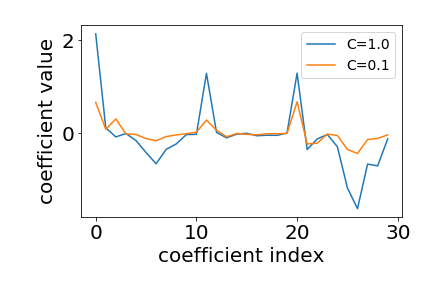Here we evaluate $20$ parameter values for $C$ using cross validation. We then use the roc_auc_score to compare the predictive accuracy across the various regularization parameters as follows:

In :
a = np.linspace(0, 1, 20)
b = [0.5]
Cs = np.sort(np.concatenate((a, b)))

enet_result, enet_coeffs = pd.DataFrame(), pd.DataFrame()
for i, C in enumerate(Cs):
print(C)
coeffs, test_results = [], []
lr_enet = LogisticRegression(penalty='elasticnet', solver='saga', l1_ratio=C, random_state=22, n_jobs=-1)
for train, test in cv.split(X.values, y.values):

X_train0 = X.iloc[train]
y_train0 = y.iloc[train]
lr_enet.fit(X_train0, y_train0)
coeffs.append(np.squeeze(lr_enet.coef_))

X_test0 = X.iloc[test]
y_test0 = y.iloc[test]
y_prob0 = lr_enet.predict_proba(X_test0)[:,1]

auc = roc_auc_score(y_test0, y_prob0)

test_results.append([auc, C])

test_results = pd.DataFrame(test_results, columns=['auc', 'C'])
enet_result = enet_result.append(test_results)
enet_coeffs[C] = np.mean(coeffs, axis=0)

0.0
0.05263157894736842
0.10526315789473684
0.15789473684210525
0.21052631578947367
0.2631578947368421
0.3157894736842105
0.3684210526315789
0.42105263157894735
0.47368421052631576
0.5
0.5263157894736842
0.5789473684210527
0.631578947368421
0.6842105263157894
0.7368421052631579
0.7894736842105263
0.8421052631578947
0.894736842105263
0.9473684210526315
1.0

In :
enet_result.describe()

Out:
auc C
count 63.000000 63.000000
mean 0.719001 0.500000
std 0.156965 0.298553
min 0.260870 0.000000
25% 0.658027 0.263158
50% 0.676667 0.500000
75% 0.876254 0.736842
max 0.939799 1.000000

We can again plot the accuracy result for the range of hyperparameter values alongside the coefficient path that shows the improvements in predictive accuracy as the coefficients are a bit shrunk at the optimal regularization value $10^4$:

In :
fig, axes = plt.subplots(ncols=2, sharex=True, figsize=(12,6))

best_C = enet_result.groupby('C').auc.mean().idxmax()
best_accuracy = enet_result.groupby('C').auc.mean().max()
mean_auc = enet_result.groupby('C').auc.mean().median()

enet_result.groupby('C')['auc'].mean().plot(logx=True, title='AUC Scores', ax=axes,
label=f'Best AUC = {round(best_accuracy,3)}')
axes.axhline(mean_auc, color='r', label=f'Mean AUC = {mean_auc}', ls='--')
axes.axvline(x=best_C, c='darkgrey', ls='--', label=f'Best C = {best_C}')
axes.set_xlabel('Regularization')
axes.set_ylabel('AUC')
axes.legend(loc='best')

enet_coeffs.T.plot(legend=False, logx=True, title='Elasticnet Path', ax=axes)
axes.set_xlabel('Regularization')
axes.set_ylabel('Coefficients')
axes.axvline(x=best_C, c='darkgrey', ls='--')
fig.tight_layout();